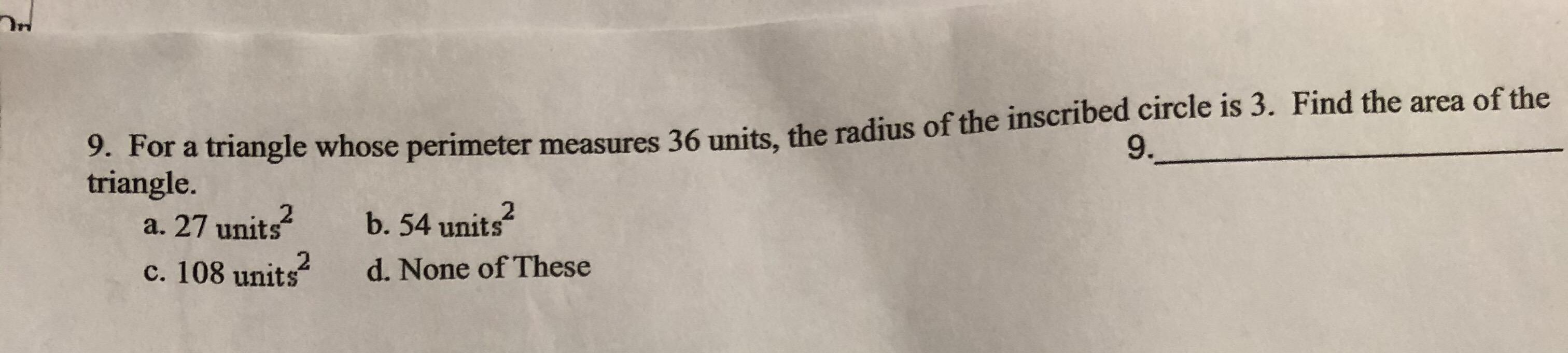# 9. For a triangle whose perimeter measures 36 units, the radius of the inscribed circle is 3. Find the area of the9.Mtriangle.a. 27 unitsc. 108 unitsb. 54 unitsd. None of These

Question
6 viewshelp_outlineImage Transcriptionclose9. For a triangle whose perimeter measures 36 units, the radius of the inscribed circle is 3. Find the area of the 9.M triangle. a. 27 units c. 108 units b. 54 units d. None of These fullscreen
check_circle

Step 1

It is given that, the perimeter of triangle is 36 units and radius of inscribed circle is 3 units.

Step 2

To Find: The area of triangle.

Step 3

The perimeter of triangle is 36 units.

Therefore, semi-...

### Want to see the full answer?

See Solution

#### Want to see this answer and more?

Solutions are written by subject experts who are available 24/7. Questions are typically answered within 1 hour.*

See Solution
*Response times may vary by subject and question.
Tagged in

### Geometry